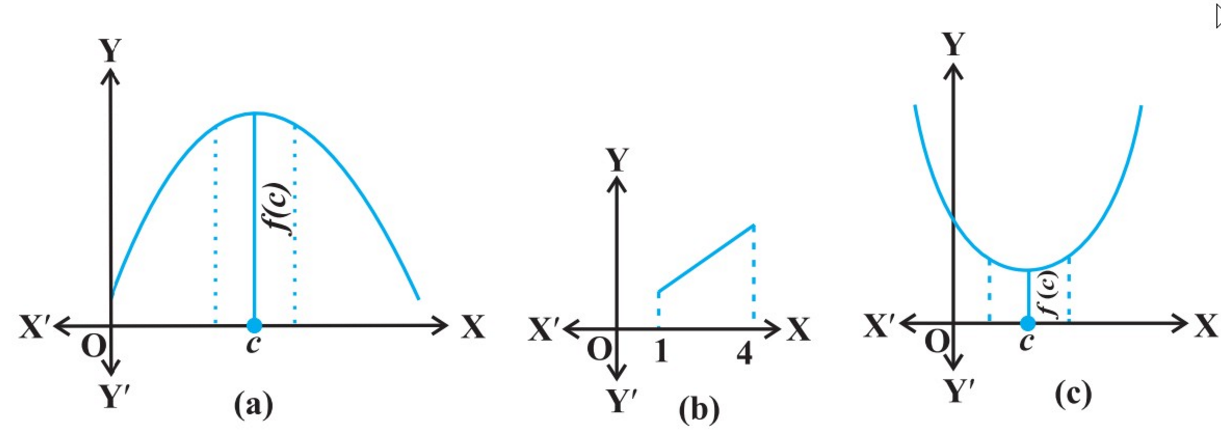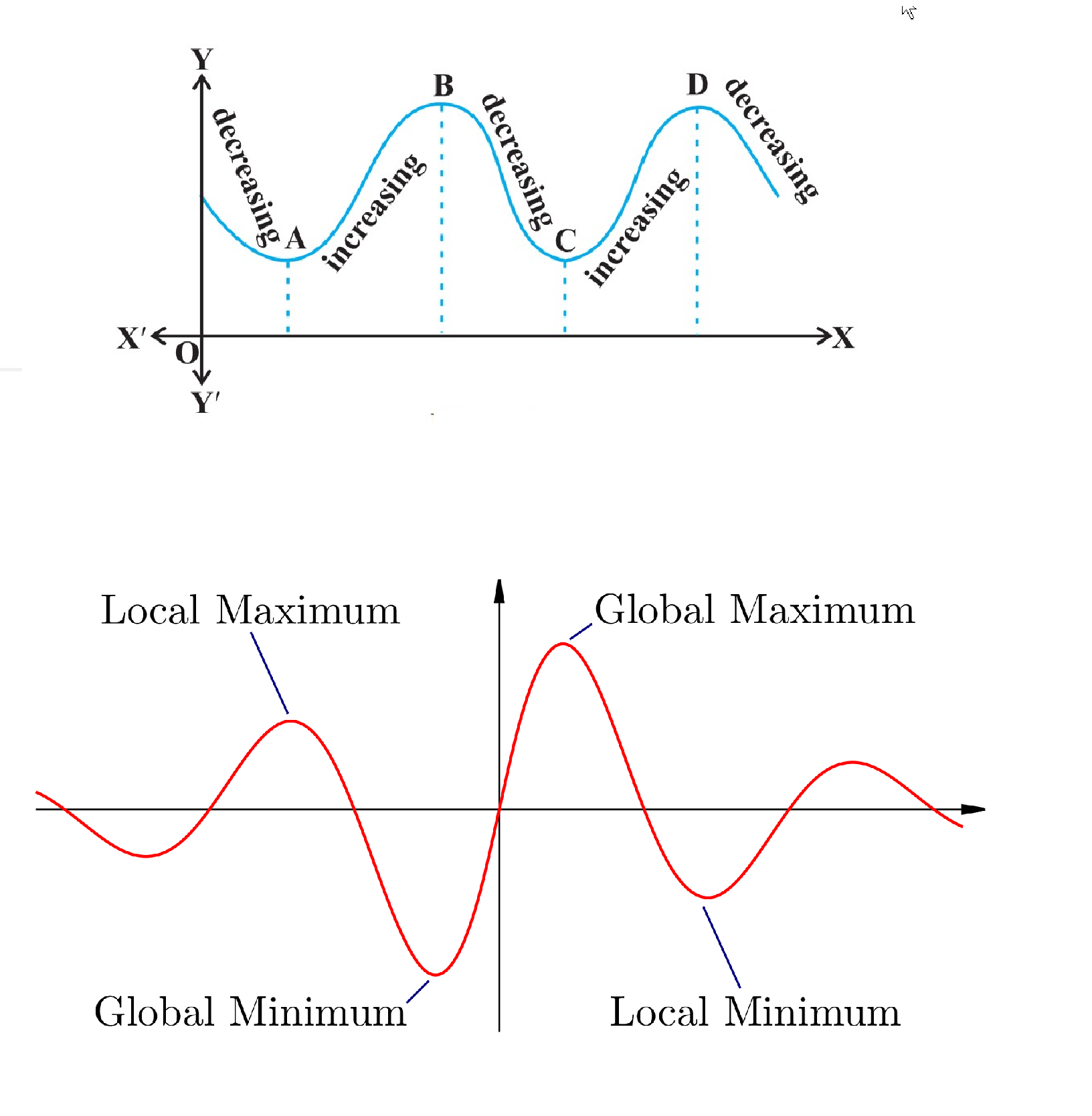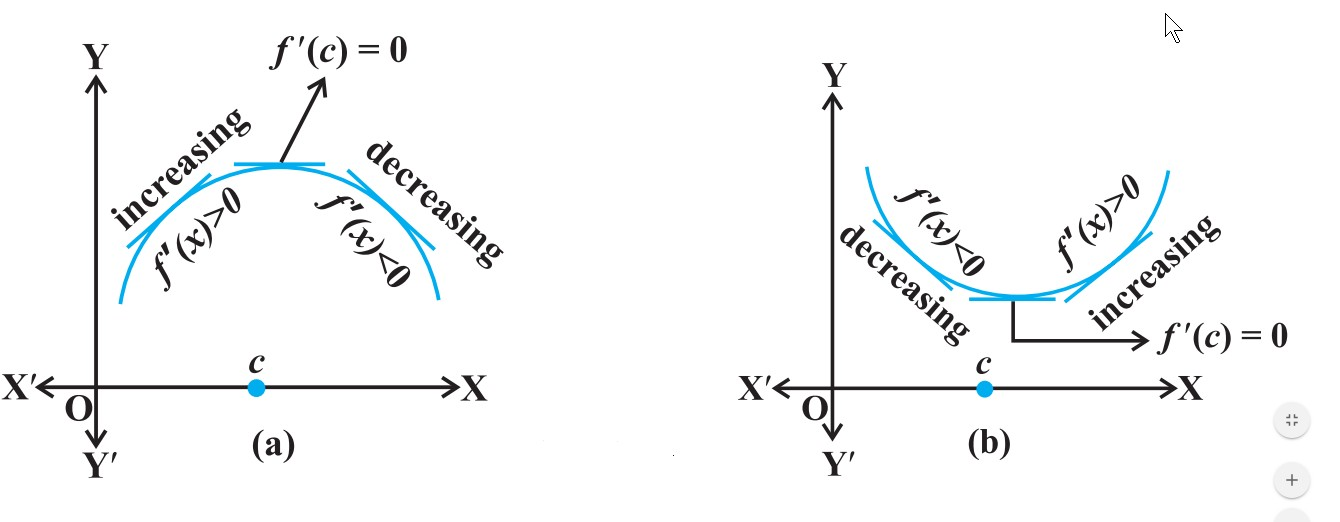Mathematics Maxima and Minima For CBSE-NCERT
Click for Only Video

### Topic covered

star Maxima and Minima

star Local maximum value and local minimum value

### Maxima and Minima\color{green} ✍️ Let f be a function defined on an interval I.

(a) Then f is said to have a maximum value in I, if there exists a point c in I such that

color{green}{f (c) ≥ f (x)} , for all x ∈ I.

=> The number f(c) is called the maximum value of f in I and the point c is called a point of maximum value of f in I.

(b) f is said to have a minimum value in I, if there exists a point c in I such that

color{green}{f (c) ≤ f (x)}, for all x ∈ I.

=> The number f(c), in this case, is called the minimum value of f in I and the point c, in this case, is called a point of minimum value of f in I.

\color{green} ✍️ (c) f is said to have an extreme value in I if there exists a point c in I such that f (c) is either a maximum value or a minimum value of f in I.

\color{green} ✍️ The number f (c), in this case, is called an extreme value of f in I and the point c is called an extreme point.

Q 3135145962Find the maximum and the minimum values, if any, of the function f given by

f (x) = x^2, x ∈ R .
Class 12 Chapter 6 Example 26Solution:

From the graph of the given function (Fig 6.10),
we have f (x) = 0 if x = 0. Also

f (x) ≥ 0, for all x ∈ R.

Therefore, the minimum value of f is 0 and the point
of minimum value of f is x = 0. Further, it may be observed
from the graph of the function that f has no maximum
value and hence no point of maximum value of f in R.

Note : If we restrict the domain of f to [– 2, 1] only, then f will have maximum value (– 2)^2 = 4 at x = – 2.
Q 3165145965Find the maximum and minimum values
of f , if any, of the function given by f (x) = | x |, x ∈ R.
Class 12 Chapter 6 Example 27Solution:

From the graph of the given function
(Fig 6.11) , note that

f (x) ≥ 0, for all x ∈ R and f (x) = 0 if x = 0.
Therefore, the function f has a minimum value 0
and the point of minimum value of f is x = 0. Also, the
graph clearly shows that f has no maximum value in
R and hence no point of maximum value in R.

Note : (i) If we restrict the domain of f to [– 2, 1] only, then f will have maximum value |– 2| = 2.
(ii) One may note that the function f in Example is not differentiable at x = 0
Q 3115145969Find the maximum and the minimum values, if any, of the function
given by

f (x) = x, x ∈ (0, 1).
Class 12 Chapter 6 Example 28Solution:

The given function is an increasing (strictly) function in the given interval
(0, 1). From the graph (Fig 6.12) of the function f , it
seems that, it should have the minimum value at a
point closest to 0 on its right and the maximum value
at a point closest to 1 on its left. Are such points
available? Of course, not. It is not possible to locate
such points. In fact, if a point x_0 is closest to 0, then

we find  (x_0)/2 < x_0 for all x_0 ∈ (0,1)  . Also, if x_1 is

closest to 1, then  (x_1 +1)/2 > x_1 for all x_1 ∈(0,1) .

Therefore, the given function has neither the maximum value nor the minimum
value in the interval (0,1).

"Remark:" The reader may observe that in Example 28, if we include the points 0 and 1 in the domain of f , i.e., if we extend the domain of f to [0,1], then the function f has minimum value 0 at x = 0 and maximum value 1 at x = 1. Infact, we have the following results (The proof of these results are beyond the scope of the present text)

### Monotonic Functions

=> By a monotonic function f in an interval I, we mean that f is either increasing in I or decreasing in I.

=>color{green}{"Every monotonic function assumes its maximum/minimum value at the"}
color{green}{" end points of the domain of definition of the function."}

=> Every continuous function on a closed interval has a maximum and a minimum value.

### Local/Global maximum value and Local/Global minimum value=> Observe that at points A, B, C and D on the graph, the function changes its nature from decreasing to increasing or vice-versa. These points may be called turning points of the given function.

=> The function has maximum value in some neighbourhood of points B and D which are at the top of their respective hills. For this reason, the points A and C may be regarded as points of local minimum value (or relative minimum value) and points B and D may be regarded as points of local maximum value (or relative maximum value) for the function

\color{green} ✍️ Local maxima and local minima are the largest and smallest value of the function, either within a given range (the local or relative extrema) or in an entire domain of a function .

\color{green} ✍️ Global maxima and Global minima are the largest and smallest value of the function, either within a given range or entire domain of a function

See Fig =>

### Concept Of Local minima and local maxima\color{green} ✍️ Let f be a real valued function and let c be an interior point in the domain of f.

(a) c is called a point of local maxima if there is an h > 0 such that

color{red}{f (c) ≥ f (x)}, for all x in (c – h, c + h)

=> The value f (c) is called the local maximum value of f.

(b) =>c is called a point of local minima if there is an h > 0 such that

color{red}{f (c) ≤ f (x),} for all x in (c – h, c + h)

=> The value f (c) is called the local minimum value of f .

color{blue}{"Note :"} The function f is increasing (i.e., (f ′(x) > 0) in the interval (c – h, c) and decreasing (i.e., (f ′(x) < 0) in the interval (c, c + h).

=>Similarly, if c is a point of local minima of f , then the graph of f around c will be as shown in fig.

Here f is decreasing (i.e., f ′(x) < 0) in the interval (c – h, c) and increasing (i.e., (f ′(x) > 0) in the interval (c, c + h).

### Theorem\color{green} ✍️ Let f be a function defined on an open interval I. Suppose c ∈ I be any point.
If f has a local maxima or a local minima at x = c, then either f ′(c) = 0 or color{red}{f \ \ "is not differentiable at" \ \ c.}

color{blue}{"Remark-"} The converse of above theorem need not be true, that is, a point at which the derivative vanishes need not be a point of local maxima or local minima.

=> For example, if f (x) = x^3, then f′(x)= 3x^2 and so f′(0) = 0. But 0 is neither a point of local maxima nor a point of local minima .

### Summery